# How to Print tuple's elements in PythonLast updated: Feb 21, 2023
5 min## #Print tuple elements in Python

Use the `print()` function to print a tuple in Python, e.g. `print(my_tuple)`.

If the value is not of type tuple, use the `tuple()` class to convert it to a tuple and print the result, e.g. `tuple([1, 2])`.

main.py
```Copied!```my_tuple = ('one', 'two', 'three')

# ✅ print a tuple
print(my_tuple)  # 👉️ ('one', 'two', 'three')

# ✅ print the first element in a tuple
print(my_tuple)  # 👉️ 'one'

# ✅ print the last element in a tuple
print(my_tuple[-1])  # 👉️ 'three'

# ✅ print a slice of a tuple
print(my_tuple[0:2])  # 👉️ ('one', 'two')
``````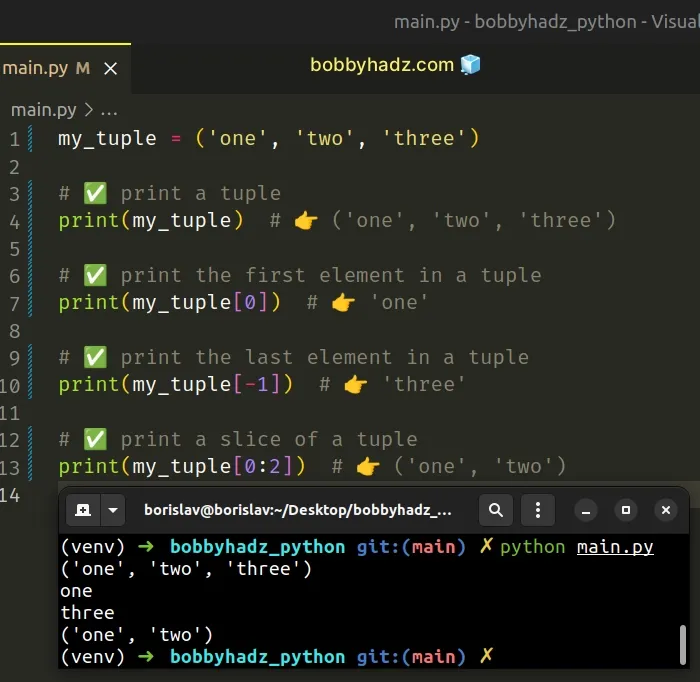We used the `print()` function to print a tuple's elements.

The print function takes one or more objects and prints them to `sys.stdout`.

If you have a tuple, you can pass it directly to the `print()` function to print it.

main.py
```Copied!```my_tuple = ('one', 'two', 'three')

print(my_tuple)  # 👉️ ('one', 'two', 'three')
``````

## #Printing a specific tuple element or slice

If you need to print an element in the tuple, access the element at its specific index.

main.py
```Copied!```my_tuple = ('one', 'two', 'three')

# ✅ print the first element in a tuple
print(my_tuple)  # 👉️ 'one'

# ✅ print the last element in a tuple
print(my_tuple[-1])  # 👉️ 'three'

# ✅ print a slice of a tuple
print(my_tuple[0:2])  # 👉️ ('one', 'two')
``````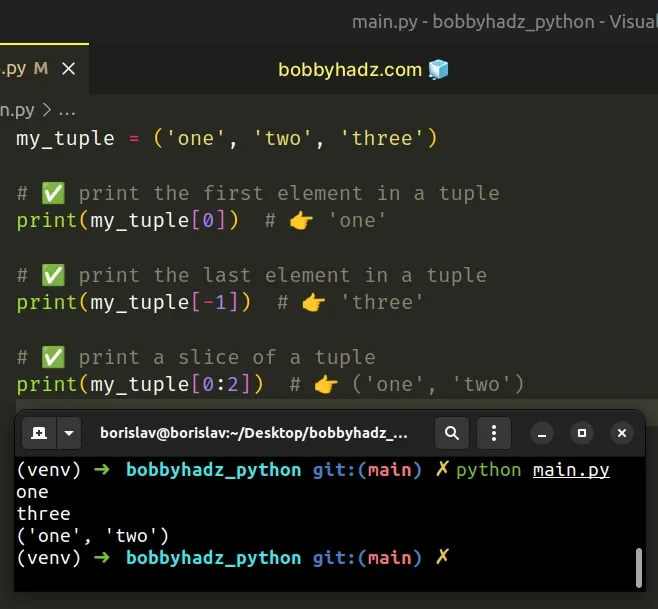The syntax for tuple slicing is `my_tuple[start:stop:step]`.

The `start` index is inclusive and the `stop` index is exclusive (up to, but not including).

main.py
```Copied!```my_tuple = ('one', 'two', 'three')

print(my_tuple[0:2]) # 👉️ ('one', 'two')
print(my_tuple[1:3]) # 👉️ ('two', 'three')
``````
Python indexes are zero-based, so the first element in a tuple has an index of `0`, and the last element has an index of `-1` or `len(my_tuple) - 1`.

## #Print a tuple without parentheses in Python

Use the `str.join()` method to print a tuple without parentheses.

The `str.join()` method will return a string containing the tuple's elements without parentheses, with a comma separator.

main.py
```Copied!```# ✅ print a tuple of strings without parentheses

tuple_of_str = ('one', 'two', 'three')

result = ','.join(tuple_of_str)
print(result)  # 👉️ 'one,two,three'
``````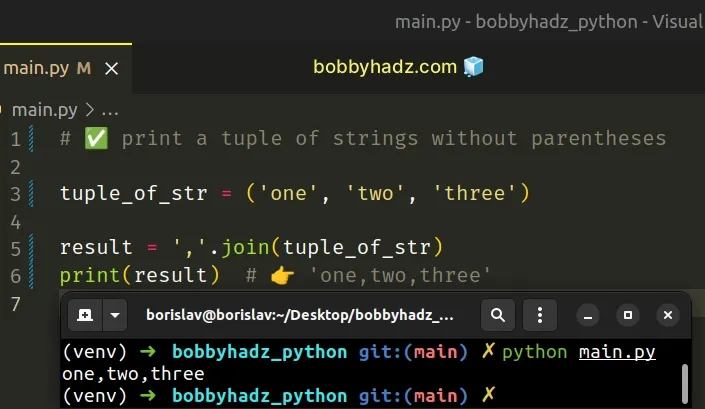We used the `str.join()` method to print a tuple without parentheses.

The str.join method takes an iterable as an argument and returns a string which is the concatenation of the strings in the iterable.

## #Print a tuple of integers without parentheses

Note that the method raises a `TypeError` if there are any non-string values in the iterable.

If your tuple contains numbers or other types, convert all of the values to string before calling `join()`.

main.py
```Copied!```tuple_of_int = (1, 2, 3)

result = ','.join(str(item) for item in tuple_of_int)
print(result)  # 👉️ '1,2,3'
``````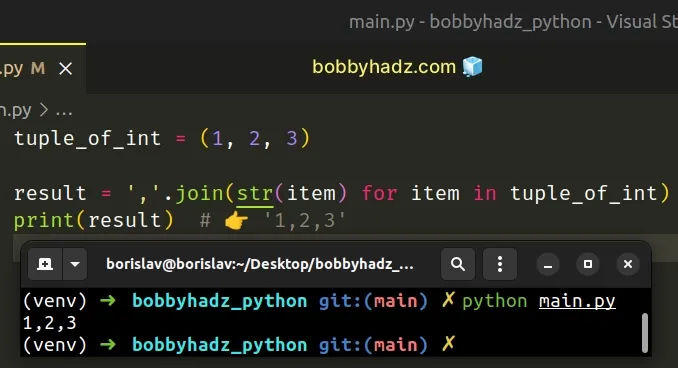The example uses a generator expression to convert each integer in the tuple to a string.

Generator expressions are used to perform some operation for every element or select a subset of elements that meet a condition.

The string the `join()` method is called on is used as the separator between the elements.

main.py
```Copied!```my_tuple = ('one', 'two', 'three')

my_str = ', '.join(my_tuple)
print(my_str)  # 👉️ "one, two, three"
``````

If you don't need a separator and just want to join the iterable's elements into a string, call the `join()` method on an empty string.

main.py
```Copied!```my_tuple = ('one', 'two', 'three')

my_str = ''.join(my_tuple)
print(my_str)  # 👉️ "onetwothree"
``````

If you need to print the tuple's elements without parentheses and separated by spaces, call the `str.join()` method on a string containing a space.

main.py
```Copied!```my_tuple = ('one', 'two', 'three')

my_str = ' '.join(my_tuple)
print(my_str)  # 👉️ "one two three"
``````

## #Print a list of tuples without brackets and parentheses

If you need to print a list of tuples without brackets and parentheses, use 2 calls to the `str.join()` method.

main.py
```Copied!```list_of_tuples = [(1, 2), (3, 4), (5, 6)]

result = ','.join(','.join(str(item) for item in tup)
for tup in list_of_tuples)

print(result)  # 👉️ '1,2,3,4,5,6'
``````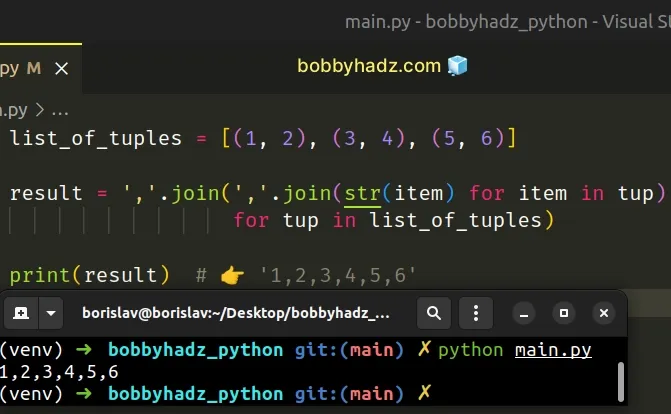The inner call to the `join()` method joins the items of the tuple of the current iteration.

We used the str() class to convert each number to a string.

The last step is to use the `join()` method to join the tuples in the list into a string with a comma separator.

## #Print a tuple with string formatting in Python

Use a formatted string literal to print a tuple with string formatting.

main.py
```Copied!```my_tuple = ('a', 'b', 'c')

result = f'Example tuple: {my_tuple}'
print(result)  # 👉️ Example tuple: ('a', 'b', 'c')
``````

The example uses a formatted string literal to print a tuple with string formatting.

Formatted string literals (f-strings) let us include expressions inside of a string by prefixing the string with `f`.
main.py
```Copied!```my_str = 'is subscribed:'
my_bool = True

result = f'{my_str} {my_bool}'
print(result)  # 👉️ is subscribed: True
``````

Make sure to wrap expressions in curly braces - `{expression}`.

You can use bracket notation to access a tuple element at an index.

main.py
```Copied!```my_tuple = ('a', 'b', 'c')

result = f'first: {my_tuple}, second: {my_tuple}, third: {my_tuple}'
print(result)  # 👉️ first: a, second: b, third: c
``````
Python indexes are zero-based, so the first element in a tuple has an index of `0`, and the last element has an index of `-1` or `len(my_tuple) - 1`.

## #Print a tuple with string formatting using `str.format()`

Alternatively, you can use the `str.format()` method.

main.py
```Copied!```my_tuple = ('a', 'b', 'c')

result = 'Example tuple: {}'.format(my_tuple)
print(result)  # 👉️ Example tuple: ('a', 'b', 'c')

result = 'first: {}, second: {}, third: {}'.format(*my_tuple)
print(result)  # 👉️ first: a, second: b, third: c
``````

The str.format method performs string formatting operations.

The string the method is called on can contain replacement fields specified using curly braces `{}`.

Make sure to provide exactly as many arguments to the `format()` method as you have replacement fields in the string.

You can use the iterable unpacking operator to unpack the tuple's elements in the call to the `format()` method if you need to access them in the string.

main.py
```Copied!```my_tuple = ('a', 'b', 'c')

result = 'first: {}, second: {}, third: {}'.format(*my_tuple)
print(result)  # 👉️ first: a, second: b, third: c
``````

The * iterable unpacking operator enables us to unpack an iterable in function calls, in comprehensions and in generator expressions.

The iterable unpacking operator unpacks the tuple and passes its elements as multiple, comma-separated arguments in the call to the `str.format()` method.